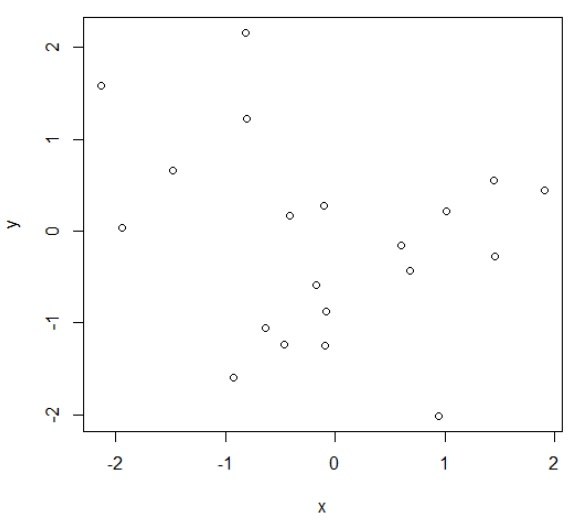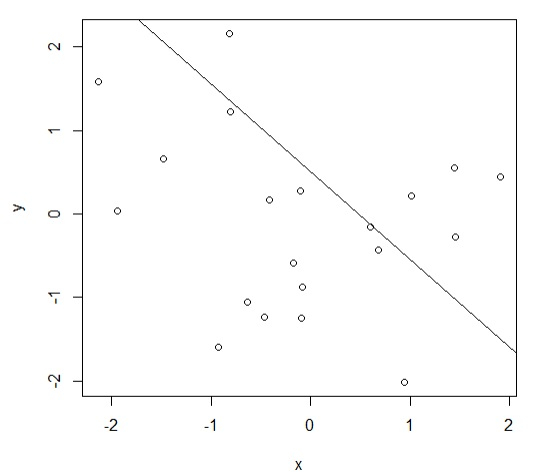# How to add a regression line to a plot in base R if intercept and slope are given?

To add a regression line to a plot in base R if intercept and slope are given, we can follow the below steps −

• First of all, create two vectors and the scatterplot between them.
• Then, use abline function to create the regression line with intercept and slope given by a and b respectively.

## Create the vectors and scatterplot

Use plot functions to create scatterplot between two random vectors x and y −

Live Demo

> x<-round(rnorm(20),2)
> y<-round(rnorm(20),2)
> plot(x,y)

## Output## Add regression line with given intercept and slope

#### Example

Using abline function to add the regression line to the scatterplot with given intercept a = 0.51 and slope = -1.05 −

Live Demo

> x<-round(rnorm(20),2)
> y<-round(rnorm(20),2)
> plot(x,y)
> abline(a=0.51,b=-1.05)

## Output